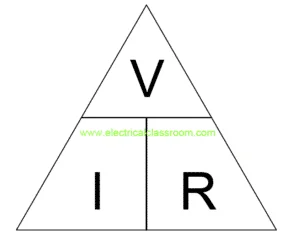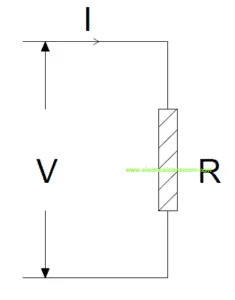# Ohm’s Law – Relationship between current and voltage

Ohm’s law is one of the basic principles of electricity. It relates the basic parameters of electricity, Current and voltage, to each other. Georg Ohm, after whom the law was named, conduct a few experiments on circuits containing different lengths of wires and found that the voltage applied and current are directly proportional. He derived a complex equation and published it along with his results in the book Die galvanische Kette, mathematisch bearbeitet in 1827.

## What does Ohm’s law state?

Ohm’s Law states that current (I) flowing through a conductor is directly proportional to the voltage applied (V) across it, i.e.

V α I

Simply, V = IR

Where R is a constant of proportionality called resistance, which defines the resistance offered by the material of the conductor to the flow of current through it.

Total Resistance offered by a conductor to the flow of electric current depends on its length, area of cross-section and resistivity of the conductor.

#### R = ρl/A

Where ρ is the resistivity of the conductor, l is the length and A is the area of cross-section.

## Ohm’s Law for AC Circuits

In the case of AC circuits, voltage is related to current by a constant of proportionality Z (impedance) and constant of proportionality R for purely resistive circuits where (Z=R).

#### Where Z =  √[R2+X2]

Impedance Z is the total opposition offered by the circuits to AC current. It consists of a real part (resistance) and an imaginary part (Reactance).

## Ohm’s Law in Circuit Analysis

Ohm’s Law serves as the basic principle in circuit analysis. It is applicable only for linear circuits in which voltage is directly proportional to it. From the relation given by Ohm, three equations can be derived:

#### These three relations can be illustrated in a triangular for as below:From this, we can find the third parameter if any two are known.

## Unit of resistance

Unit of resistance is Ohm (Ω). One ohm is equal to the resistance offered by a material when a current of one ampere flows through it with a potential difference of one volt applied across it.

## Examples for Ohm’s LawLet us work out the following example.
The current flowing through the circuit is I = 2A and the resistance offered by the circuit to the flow of current is R = 5ohms.

Then the voltage drop across the circuit shall be 2A X 5 ohms = 10V

Similarly,

If the voltage across the resistor is V = 20V and the current flowing through it is I = 10A, then the value of resistance is 20V/10A = 2ohms.

Hence, it can be noted that current flow through a circuit depends on its resistance and the voltage applied across it.

Sharing is caring